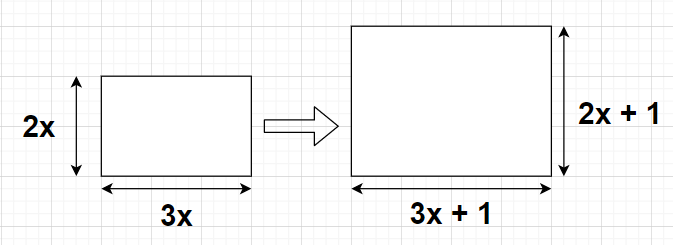QuestionAnswers

# The length and breadth of a rectangle are in the ratio 3 : 2 respectively. If the sides of the rectangle are extended on each side by 1 m, the ratio of the length to the breadth becomes 10 : 7. Find the area of the original rectangle in square metres.${\text{A}}{\text{. 23 }}{{\text{m}}^2} \\ {\text{B}}{\text{. 11 }}{{\text{m}}^2} \\ {\text{C}}{\text{. 54 }}{{\text{m}}^2} \\ {\text{D}}{\text{. 10 }}{{\text{m}}^2} \\$Hint: Here, we will proceed by obtaining one equation in one variable according to the problem statement in order to find the value of that variable. Here, we will also use the formula i.e., Area of the rectangle = (Length)(Breadth).

Given, Ratio of the original length to the original breadth of the rectangle = 3 : 2
i.e., $\dfrac{{{\text{Original Length}}}}{{{\text{Original Breadth}}}} = \dfrac{3}{2} = \dfrac{{3x}}{{2x}}$ (say)
$\Rightarrow$Original Length of the rectangle = 3x m
$\Rightarrow$Original Breadth of the rectangle = 2x m
Now, when all the sides of the rectangle are extended by 1 m, we have
Final Length of the rectangle = (3x+1) m
Final Breadth of the rectangle = (2x+1) mSo, $\dfrac{{{\text{Final Length}}}}{{{\text{Final Breadth}}}} = \dfrac{{3x + 1}}{{2x + 1}}{\text{ }} \to {\text{(1)}}$
It is given that the ratio of the final length to the final breadth of the rectangle after extending each side by 1 m is 10 : 7
i.e., $\dfrac{{{\text{Final Length}}}}{{{\text{Final Breadth}}}} = \dfrac{{10}}{7}$
By substituting equation (1) in the above equation, we get
$\Rightarrow \dfrac{{3x + 1}}{{2x + 1}} = \dfrac{{10}}{7}$
By cross multiplying the above equation, we get
$\Rightarrow 7\left( {3x + 1} \right) = 10\left( {2x + 1} \right) \\ \Rightarrow 21x + 7 = 20x + 10 \\ \Rightarrow 21x - 20x = 10 - 7 \\ \Rightarrow x = 3 \\$
$\Rightarrow$Original Length of the rectangle = 3x = 3(3) = 9 m
$\Rightarrow$Original Breadth of the rectangle = 2x = 2(3) = 6 m
As we know that the area of any rectangle is given by
Area of the rectangle = (Length)(Breadth)
$\Rightarrow$Area of the original rectangle = (Original Length of the rectangle)(Original Breadth of the rectangle)
$\Rightarrow$Area of the original rectangle = (9)(6) = 54 ${{\text{m}}^2}$.
Hence, option C is correct.

Note: In this particular problem, the ratio given are in the most simplified form so in order to obtain the values of the numerator and denominator of that ratio we will multiply both the numerator and denominator with same number (in this case it is x) which is the same ratio as the given ratio because this number can be cancelled.
View Notes
The Perimeter of Rectangle FormulaWhat are the Functions of the Human Skeletal System?Relation Between the Length of a Given Wire and Tension for Constant Frequency Using SonometerWhat are the Successor and Predecessor?The Making of a Scientist2D and 3D FiguresChanging the Period of a PendulumSignificance of Genetics in the Process of EvolutionNCERT Book for Class 9 Social Science India And The Contemporary World 1 PDF in HindiProductivity in the EcosystemImportant Questions for CBSE Class 7 English An Alien Hand Chapter 9 - A Tiger In The HouseImportant Questions for CBSE Class 6 English A Pact with The Sun Chapter 6 - The Monkey and the CrocodileImportant Questions for CBSE Class 7 Social Science - Social and Political Life Chapter 8 - A Shirt In The MarketImportant Questions for CBSE Class 6 English A Pact with The Sun Chapter 9 - What Happened to The ReptilesImportant Questions for CBSE Class 6 English A Pact with The Sun Chapter 8 - A Pact with the SunImportant Questions for CBSE Class 9 Science Chapter 4 - Structure of The AtomImportant Questions for CBSE Class 6 Social Science The Earth Our Habitat Chapter 1 - The Earth In The Solar SystemImportant Questions for CBSE Class 6 English A Pact with The Sun Chapter 2 - The Friendly MongooseImportant Questions for CBSE Class 7 Social Science Our Environment Chapter 9 - Life in The DesertsImportant Questions for CBSE Class 9 Maths Chapter 9 - Areas of Parallelograms and TrianglesCBSE Class 10 Hindi A Question Paper 2020Hindi A Class 10 CBSE Question Paper 2009Hindi A Class 10 CBSE Question Paper 2015Hindi A Class 10 CBSE Question Paper 2007Hindi A Class 10 CBSE Question Paper 2013Hindi A Class 10 CBSE Question Paper 2016Hindi A Class 10 CBSE Question Paper 2012Hindi A Class 10 CBSE Question Paper 2010Hindi A Class 10 CBSE Question Paper 2008Hindi A Class 10 CBSE Question Paper 2014RD Sharma Solutions for Class 9 Maths Chapter 18 - Surface Area and Volume of a Cuboid and CubeNCERT Solutions for Class 9 Maths Chapter 9 Area Of Parrallelograms and triangles In HindiRS Aggarwal Class 9 Solutions Chapter-9 Congruence of Triangles and Inequalities in a TriangleRD Sharma Solutions for Class 9 Maths Chapter 21 - Surface Area and Volume of a SphereNCERT Solutions for Class 9 Social Science India and the Contemporary World - I Chapter 5 - Pastoralists in the Modern WorldRD Sharma Solutions for Class 9 Maths Chapter 19 - Surface Area and Volume of A Right Circular CylinderRD Sharma Solutions for Class 9 Maths Chapter 20 - Surface Area and Volume of A Right Circular ConeNCERT Solutions for Class 9 Science Chapter 4 Structure of The Atom in HindiNCERT Solutions for Class 9 Maths Chapter 9 Areas of Parallelograms and TrianglesNCERT Solutions for Class 6 Science Chapter 9 The Living Organisms and Their Surroundings In Hindi